Ex 5.2

Chapter 5 Class 11 Complex Numbers
Serial order wise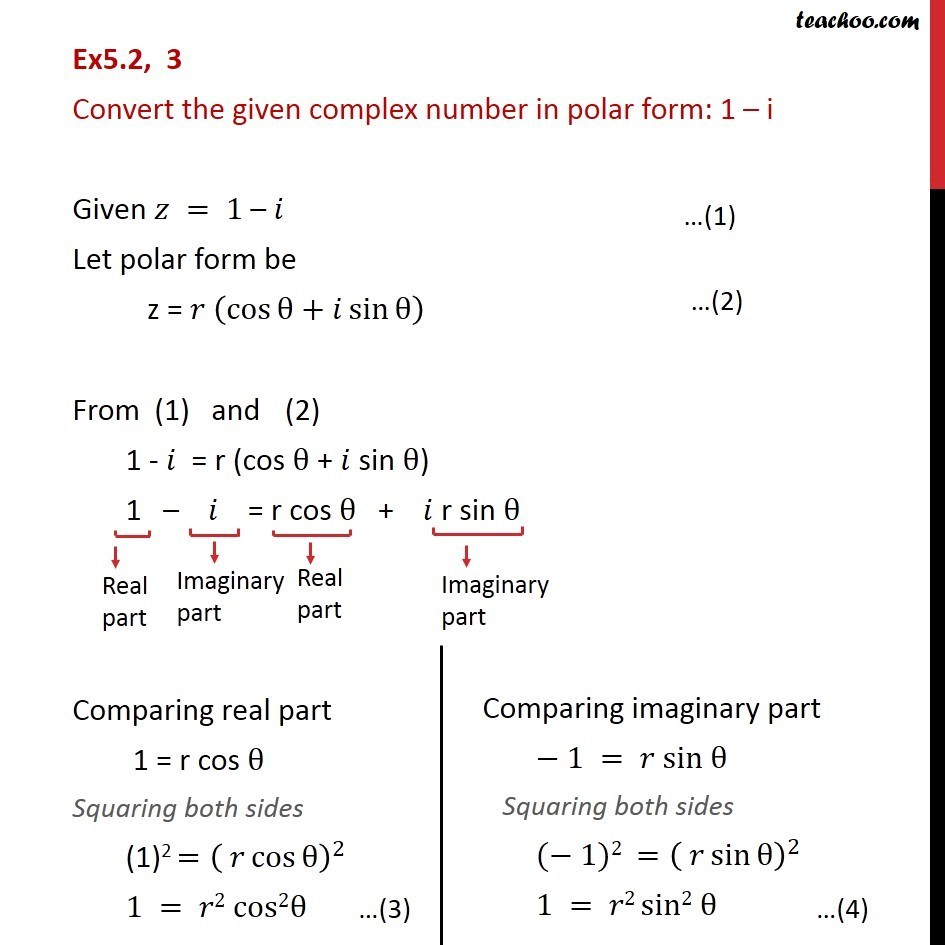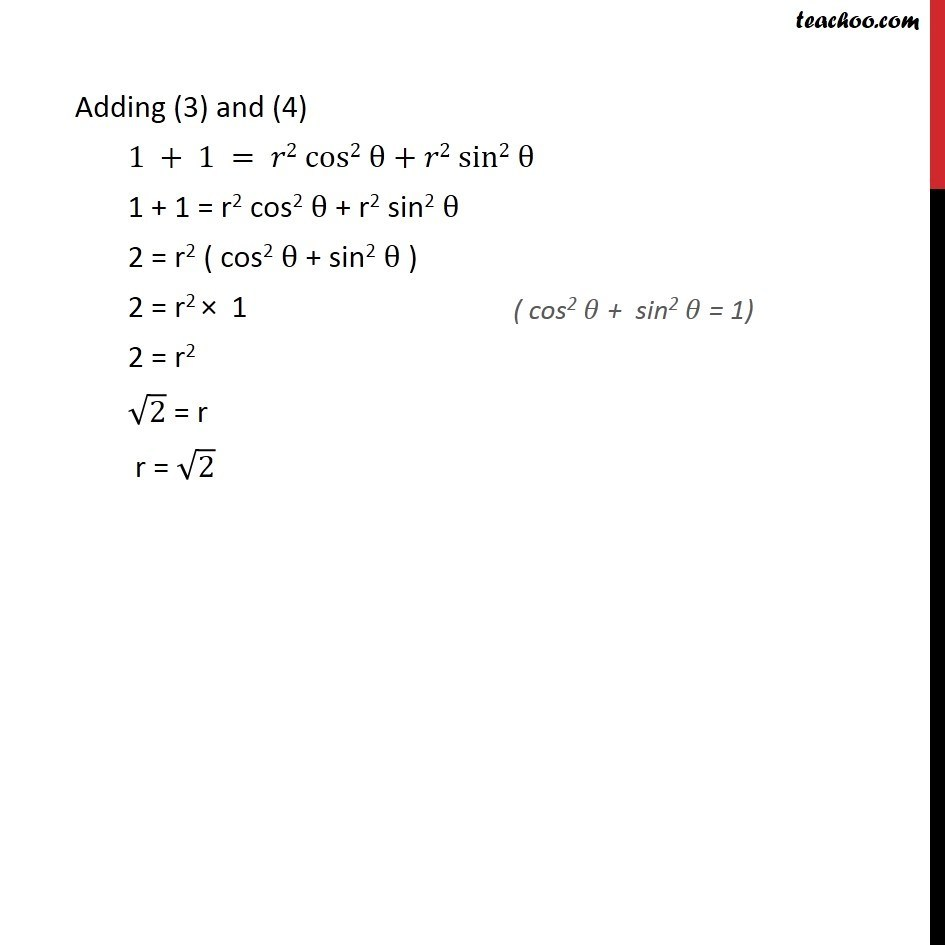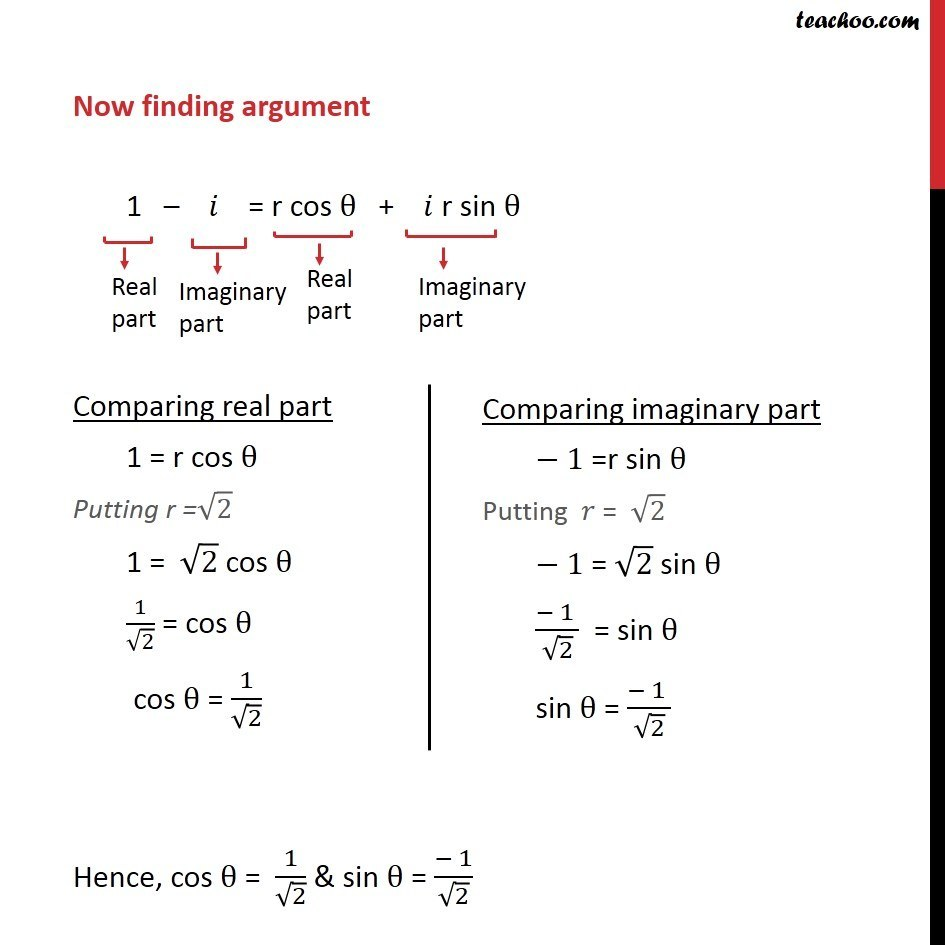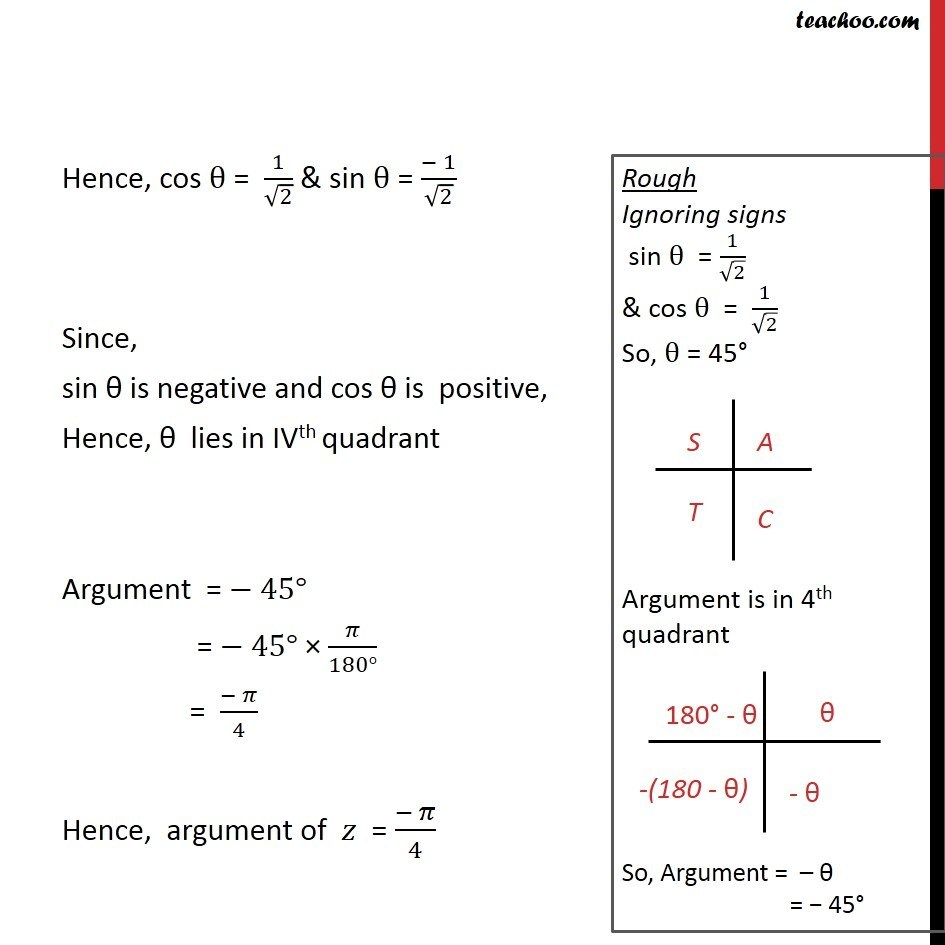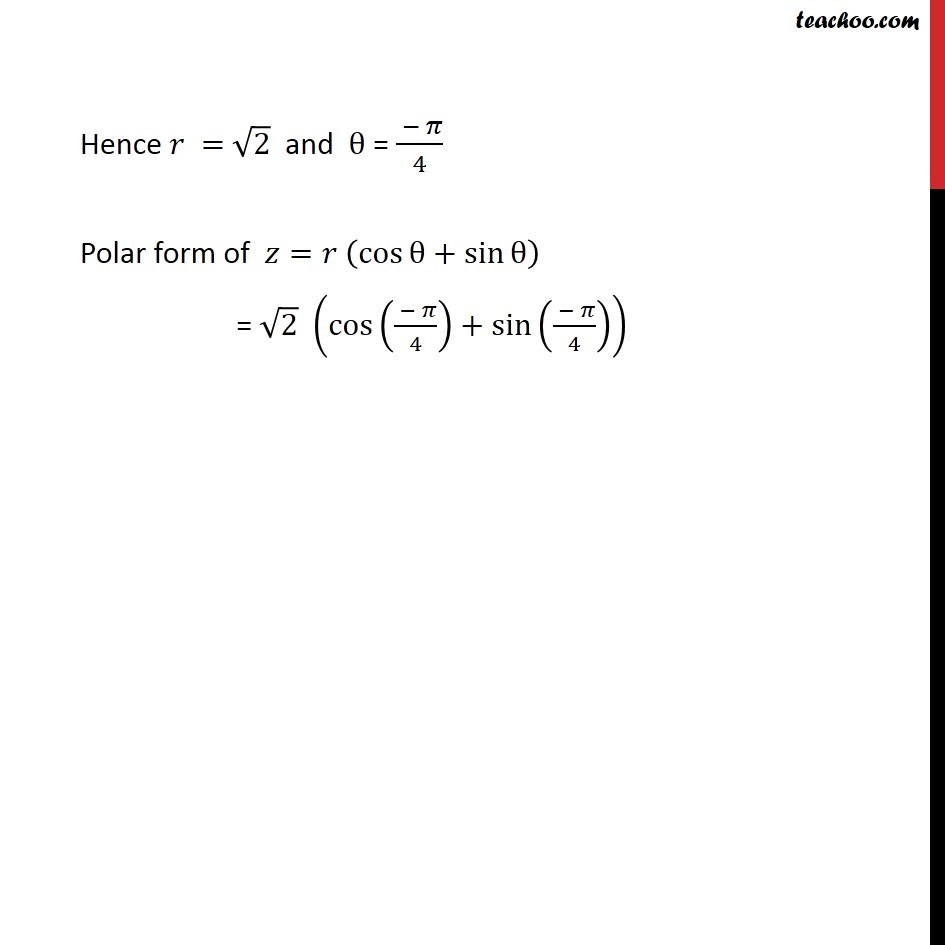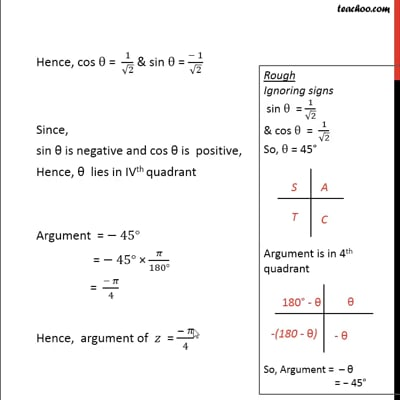This video is only available for Teachoo black users

Solve all your doubts with Teachoo Black (new monthly pack available now!)

### Transcript

Ex5.2, 3 Convert the given complex number in polar form: 1 – i Given 𝑧 = 1 – 𝑖 Let polar form be z = 𝑟 (cos⁡θ+𝑖 sin⁡θ ) From (1) and (2) 1 - 𝑖 = r (cos θ + 𝑖 sin θ) 1 – 𝑖 = r cos θ + 𝑖 r sin θ Comparing real part 1 = r cos θ Squaring both sides (1)2 =( 𝑟 cos⁡θ )^2 1 = 𝑟2 cos2θ Adding (3) and (4) 1 + 1 = 𝑟2 cos2 θ + 𝑟2 sin2 θ 1 + 1 = r2 cos2 θ + r2 sin2 θ 2 = r2 ( cos2 θ + sin2 θ ) 2 = r2 × 1 2 = r2 √2 = r r = √2 Now finding argument 1 – 𝑖 = r cos θ + 𝑖 r sin θ Comparing real part 1 = r cos θ Putting r =√2 1 = √2 cos θ 1/√2 = cos θ cos θ = 1/√2 Hence, cos θ = 1/√2 & sin θ = (− 1)/√2 Hence, cos θ = 1/√2 & sin θ = (− 1)/√2 Since, sin θ is negative and cos θ is positive, Hence, θ lies in IVth quadrant Argument = − 45° = − 45° × 𝜋/(180°) = (− 𝜋)/4 Hence, argument of 𝑧 = (− 𝜋)/4 Hence 𝑟 = √2 and θ = ( − 𝜋)/4 Polar form of 𝑧=𝑟 (cos⁡θ+sin⁡θ ) = √2 (cos(( − 𝜋)/4)+sin(( − 𝜋)/4))